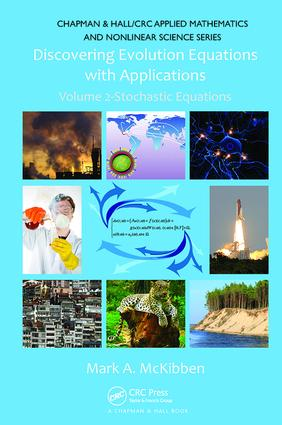# Discovering Evolution Equations with Applications

## Volume 2-Stochastic Equations, 1st Edition

Chapman and Hall/CRC

463 pages

##### Purchasing Options:\$ = USD
Paperback: 9781138113589
pub: 2017-06-14
\$82.95
x
Hardback: 9781420092110
pub: 2011-06-03
\$220.00
x
eBook (VitalSource) : 9780429142109
pub: 2011-06-03
from \$39.98

FREE Standard Shipping!

### Description

Most existing books on evolution equations tend either to cover a particular class of equations in too much depth for beginners or focus on a very specific research direction. Thus, the field can be daunting for newcomers to the field who need access to preliminary material and behind-the-scenes detail. Taking an applications-oriented, conversational approach, Discovering Evolution Equations with Applications: Volume 2-Stochastic Equations provides an introductory understanding of stochastic evolution equations.

The text begins with hands-on introductions to the essentials of real and stochastic analysis. It then develops the theory for homogenous one-dimensional stochastic ordinary differential equations (ODEs) and extends the theory to systems of homogenous linear stochastic ODEs. The next several chapters focus on abstract homogenous linear, nonhomogenous linear, and semi-linear stochastic evolution equations. The author also addresses the case in which the forcing term is a functional before explaining Sobolev-type stochastic evolution equations. The last chapter discusses several topics of active research.

Each chapter starts with examples of various models. The author points out the similarities of the models, develops the theory involved, and then revisits the examples to reinforce the theoretical ideas in a concrete setting. He incorporates a substantial collection of questions and exercises throughout the text and provides two layers of hints for selected exercises at the end of each chapter.

Suitable for readers unfamiliar with analysis even at the undergraduate level, this book offers an engaging and accessible account of core theoretical results of stochastic evolution equations in a way that gradually builds readers’ intuition.

A Basic Analysis Toolbox

Some Basic Mathematical Shorthand

Set Algebra

Functions

The Space (R, |·|)

Sequences in (R, |·|)

The Spaces (RN, ||·||RN) and (MN(R), ||·||MN(R))

Abstract Spaces

Elementary Calculus in Abstract Spaces

Some Elementary ODEs

A Handful of Integral Inequalities

Fixed-Point Theory

The Bare-Bone Essentials of Probability Theory

Formalizing Randomness

R-Valued Random Variables

Introducing the Space L2 (Ω;R)

RN-Valued Random Variables

Conditional Probability and Independence

Conditional Expectation—A Very Quick Description

Stochastic Processes

Martingales

The Wiener Process

Summary of Standing Assumptions

Linear Homogenous Stochastic Evolution Equations in R

Random Homogenous Stochastic Differential Equations

Introducing the Lebesgue and Itó Integrals

The Cauchy Problem—Formulation

Existence and Uniqueness of a Strong Solution

Continuous Dependence on Initial Data

Statistical Properties of the Strong Solution

Some Convergence Results

A Brief Look at Stability

A Classical Example

Homogenous Linear Stochastic Evolution Equations in RN

Motivation by Models

Deterministic Linear Evolution Equations in RN

Exploring Two Models

The Lebesgue and Itó Integrals in RN

The Cauchy Problem—Formulation

Existence and Uniqueness of a Strong Solution

Continuous Dependence on Initial Data

Statistical Properties of the Strong Solution

Some Convergence Results

Abstract Homogenous Linear Stochastic Evolution Equations

Linear Operators

Linear Semigroup Theory—Some Highlights

Probability Theory in the Hilbert Space Setting

Random Homogenous Linear SPDEs

Bochner and Itó Integrals

The Cauchy Problem—Formulation

The Basic Theory

Nonhomogenous Linear Stochastic Evolution Equations

Finite-Dimensional Setting

Nonhomogenous Linear SDEs in R

Nonhomogenous Linear SDEs in RN

Abstract Nonhomogenous Linear SEEs

Introducing Some New Models

Semi-Linear Stochastic Evolution Equations

Motivation by Models

Some Essential Preliminary Considerations

Growth Conditions

The Cauchy Problem

Models Revisited

Theory for Non-Lipschitz-Type Forcing Terms

Functional Stochastic Evolution Equations

Motivation by Models

Functionals

The Cauchy Problem

Models—New and Old

Sobolev-Type Stochastic Evolution Equations

Motivation by Models

The Abstract Framework

Semi-Linear Sobolev Stochastic Equations

Functional Sobolev SEEs

Beyond Volume 2

Fully Nonlinear SEEs

Time-Dependent SEEs

Quasi-Linear SEEs

McKean-Vlasov SEEs

Even More Classes of SEEs

Bibliography

Index

Guidance for Selected Exercises appears at the end of each chapter.

### About the Author/Editors

Mark A. McKibben is a professor of mathematics and computer science at Goucher College. He serves as a referee for more than 30 journals and has published numerous articles in peer-reviewed journals. Dr. McKibben earned a Ph.D. in mathematics from Ohio University. His research interests include nonlinear and stochastic evolution equations.

### Subject Categories

##### BISAC Subject Codes/Headings:
MAT003000
MATHEMATICS / Applied
MAT007000
MATHEMATICS / Differential Equations
MAT037000
MATHEMATICS / Functional Analysis